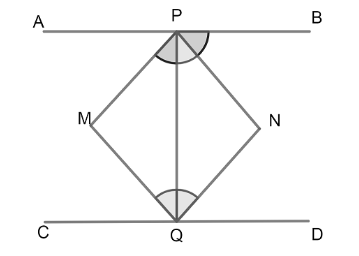Courses
Courses for Kids
Free study material
Free LIVE classes
MoreLIVE
Join Vedantu’s FREE Mastercalss

# If $APB$ and $CQD$ are two parallel lines, then bisectors of $\angle APQ$ , $\angle BPQ,\angle CQP$ and $\angle PQD$ formsA. a squareB. a rhombusC. a rectangleD. any other parallelogramVerified
335.1k+ views
Hint: First we draw two parallel lines $APB$ and $CQD$ , then, we draw angle bisectors of $\angle APQ$ , $\angle BPQ,\angle CQP$ and $\angle PQD$ . Assuming that these angle bisectors meet at point $M$ and $N$ . By joining points we draw a quadrilateral, then by observing the property of quadrilateral forms, we choose the correct answer.

Let us first draw a diagram by using information given that $APB$ and $CQD$ are two parallel lines and there are angle bisectors of $\angle APQ$ , $\angle BPQ,\angle CQP$ and $\angle PQD$ .We have given that $APB$ and $CQD$ are two parallel lines. Let us assume that the bisectors of $\angle APQ$ and $\angle CQP$ meet at point $M$ . Similarly, bisectors of $\angle BPQ$ and $\angle DQP$ meet at point $N$ .
We join $PM,QM,QN,PN$ , it forms a quadrilateral.
Now, as given $APB\parallel CQD$
So, alternate interiors angles will be equal $\angle APQ=\angle PQD$
Now, as $PM$ and $QN$ are angle bisectors of $\angle APQ$ and $\angle PQD$ respectively. So,
$\angle APM=\angle MPQ$ and $\angle BPN=\angle NPQ$
$\angle MPQ=\angle NQP$
As these are alternate interior angles so the lines $PM$ and $QN$ must be parallel.
Similarly, alternate interiors angles $\angle BPQ=\angle CQP$ are also equal.
So, the lines $PN$ and $QM$ must be parallel.
As $PM\parallel QN$ and $PN\parallel QM$ .
Opposite sides are parallel to each other, it means $PMQN$ is a parallelogram.
As $CQD$ is a straight line, so the measure of $\angle CQD=180{}^\circ$
Or we can write as $\angle CQP+\angle DQP=180{}^\circ$
Or $\angle CQM+\angle PQM+\angle DQN+\angle PQN=180{}^\circ$
As $PM$ and $QN$ are angle bisectors, So, $\angle PQM+\angle PQM+\angle PQN+\angle PQN=180{}^\circ$
$\left[ \angle CQM=\angle PQM\And \angle DQN=\angle PQN \right]$
\begin{align} & 2\angle PQM+2\angle PQN=180{}^\circ \\ & 2\left( \angle PQM+\angle PQN \right)=180{}^\circ \\ & \angle PQM+\angle PQN=\dfrac{180{}^\circ }{2} \\ & \angle PQM+\angle PQN=90{}^\circ \\ \end{align}
$\angle MQN=90{}^\circ$
So, the correct answer is “Option C”.

Note: Opposite sides of a rectangle are always equal and parallel to each other. Diagonals of a rectangle bisect each other. Measure of angles of a rectangle is equal to $90{}^\circ$. It is important to apply properties to prove it a quadrilateral. Also, applying properties is important as given in the options all are quadrilateral so we have to prove that it is a rectangle.
Last updated date: 29th Sep 2023
Total views: 335.1k
Views today: 9.35k